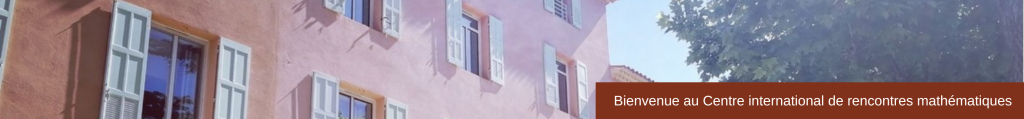GPELab, an open source Matlab toolbox for the numerical simulation of Gross-Pitaevskii equations   (pdf)

Quantized vortex stability and dynamics in superfluidity and superconductivity   (pdf)

Classical and non-classical flows of superfluids   (pdf)

High-order numerical schemes for computing the dynamics of nonlinear Schrödinger equation   (pdf)

Helicity, Topology and Kelvin Waves in reconnecting quantum knots   ﻿(pdf)﻿

• Yongyong Cai (Purdue University and Beijing Computational Science Research Center)

Ground states and dynamics  for SO coupled Bose-Einstein condensation   (pdf)

On time splitting for NLS in the semiclassical regime   (pdf)

Counterflowing superfluids   (pdf)

An overview of the BECASIM project: open source numerical simulators for the Gross-Pitaevskii equation   (pdf)

Inhomogeneities and temperature effects in Bose-Einstein condensates   (pdf)

Localization of nonlocal continuum models   (pdf)

Three-dimensional vortex structures in a rotating dipolar Bose-Einstein condensate   (pdf)

Symmetries and dynamics in a quantum-chaotic system   (pdf)

Coherent optical manipulation of plasma waves   (pdf)

Nearly parallel vortex filaments in the 3Gross-Pitaevskii equation   (pdf)

Quantum nature and statistical law in quantum turbulence   (pdf)

Superfluidity and Bogoliubov theory: rigorous results   (pdf)

A hybrid code for solving the Gross-Pitaevskii equation   (pdf)

On Some Variational Optimization Problems in Classical Fluids and Super fluids   (pdf)

Single/Multi Component Quantum Gases: Non-Equilibrum models & applications to Experiments   (pdf)

On the leapfrogging phenomenon in fluid mechanics   (pdf)

Numerical methods on simulating dynamics of the nonlinear Schrödinger equation  with rotation and/or nonlocal interactions   (pdf)

High-order commutator-free Magnus integrators for non-autonomous linear evolution equations   (pdf)

Inhomogeneous quantum turbulence in a channel   (pdf)

An efficient splitting Fourier pseudospectral method for Vlasov-Poisson-Fokker-Planck system   (pdf)

Fractional Schrödinger equation: stationary states and dynamics   (pdf)